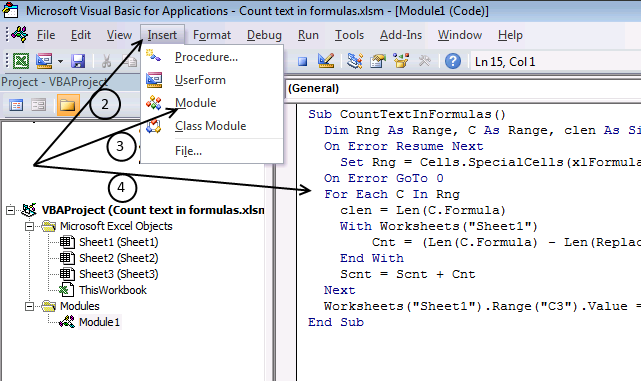Author: Oscar Cronquist Article last updated on January 09, 2018

Rahul Jadhav asks:

I have many excel files with multiple sheets and each excel sheet
has many formula which are starting from perticular word e.g. FDS, FDSB, etc some formula has FDS, FDSB occur in the middle of the formula.
i need to find out how many times FDS,FDSB has been appear in the sheet(total count)
below is the formulae for your referance
=IFERROR(FDSB(\$D21, "IC_ESTIMATE_DATE(ALL, EXP_RPT,QTR, 3, 0, , , 'MM/DD/YYYY')@RC_ESTIMATE_DATE(ALL, EXP_RPT, QTR, +3, 0, , , 'MM/DD/YYYY')"), "na")

in such a way there are many formulas in all the cells
i just need to count the how many times FDSB occur in one perticular sheet

The search string is in cell C2. Click the "start" box and the result is shown in cell c3.

In the example above, the macro counts how many times the text string "COUNTIF(" is found on the current sheet. 18 is the result. (There are 18 formulas in cell range F7:F24.)

### VBA Code

I wanted to quickly select all cells containing formulas on a sheet. I found Rick Rothstein (MVP - Excel) macro here and modified it.

```Sub CountTextInFormulas()
Dim Rng As Range, C As Range, Cnt As Double, Scnt As Double
On Error Resume Next
Set Rng = Cells.SpecialCells(xlFormulas)
On Error GoTo 0
If Not (Rng Is Nothing) Then
For Each C In Rng
With Worksheets("Sheet1")
Cnt = (Len(C.Formula) - Len(Replace(C.Formula, .Range("C2"), ""))) / Len(.Range("C2"))
End With
Scnt = Scnt + Cnt
Next
End If
Worksheets("Sheet1").Range("C3").Value = Scnt
End Sub

```

Instructions1. Go to the VB Editor (Alt+ F11)
2. Click "Insert"
3. Click "Module"
4. Paste code to module
5. Exit VB Editor

Create button

1. Go to tab "Developer"
2. Click "Insert Controls" button
3. Insert a button (Form Controls) on the sheet near cell C2.

Assign button

1. Right click on your new button
2. Click "Assign Macro..."
3. Select CountTextInFormulas
4. Click OK

### Download excel *.xlsm file

Count text in formulasv2.xlsm

### Functions in this post:

COUNTIF(range, criteria)
Counts the number of cells within a range that meet a single criterion that you specify.